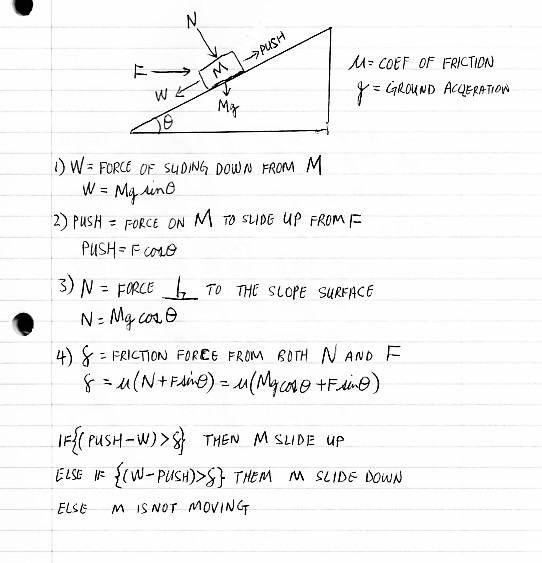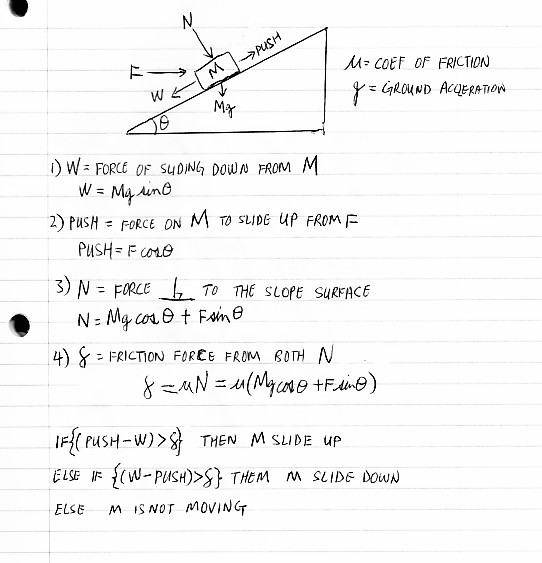# Friction problem in HS physics -- Mass moving on an inclined plane

• yungman

#### yungman

Homework Statement
Helping grand daughter
Relevant Equations
see below
This is a homework problem of my grand daughter. The question is to find out the conditions of an object M on a slope with angle shown and applied force "F". I find there are 3 conditions, sliding up, sliding down and not moving. This is my work. I just want to get comments on my work:At the bottom, I wrote out the conditions.

Thanks

Just a note: ##N## is pointing in the opposite direction of how its shown in the diagram and it needs to include the component of Force normal to the incline from the pushing force ##F##. Apply ## \sum \boldsymbol F = m \boldsymbol a ##.

EDIT: I see that you've actually done this in ##(4)## (or know this),but saying ##N = Mg \cos \theta## in ##(3)## is not correct.

Last edited:
•yungman
Homework Statement:: Helping grand daughter
Relevant Equations:: see below

This is a homework problem of my grand daughter. The question is to find out the conditions of an object M on a slope with angle shown and applied force "F". I find there are 3 conditions, sliding up, sliding down and not moving. This is my work. I just want to get comments on my work:
View attachment 314023

At the bottom, I wrote out the conditions.

Thanks
(Along with erboz's comment about the direction of the normal force.)

Taken individually your concepts are almost correct. However you are ignoring that some of these forces, such as the normal force, are a reaction to a set of applied forces. For example, if we take the +y direction to be in the direction of the normal force:
##\sum F_y = N - Mg ~ cos( \theta ) - F ~ sin( \theta )##

Then we have that ##f = \mu N##, as per its definition.

You will easily see that the normal force here is not equal to ##Mg ~ cos( \theta )##.

Now, your answers will be numerically correct... You did the Math right. But you will not have defined the normal force correctly.

It is better to apply Newton's Laws first before making generalizations.

-Dan

•erobz
Just a note: ##N## is pointing in the opposite direction of how its shown in the diagram and it needs to include the component of Force normal to the incline from the pushing force ##F##. Apply ## \sum \boldsymbol F = m \boldsymbol a ##.

EDIT: I see that you've actually done this in ##(4)## (or know this),but saying ##N = Mg \cos \theta## in ##(3)## is not correct.
I thought I represent perpendicular force to the slope surface in (3). That's the perpendicular force on the surface due to M.
In (4) I calculate the friction component part due to N. I don't understand why (3) is not correct. Please explain.

Thanks

I thought I represent perpendicular force to the slope surface in (3). That's the perpendicular force on the surface due to M.
In (4) I calculate the friction component part due to N. I don't understand why (3) is not correct. Please explain.

Thanks
The normal force is as big as it needs to be to keep the object from leaving the surface. (Else it's 0 N.) For example, if we set a book on a flat table, the net force on the book will be 0 N because it is not accelerating upward. So ##\sum F_y = N - mg = 0 \implies N = mg##. If we push down on the book with an additional force F then what is the normal force?
##\sum F_y = N - mg - F = 0 \implies N = mg + F##

The value of the size of the normal force does not have a specific equation in general. You have to find it according to the application of Newton's Laws. (Since you are also interested in friction, I might add that the static friction force has the same property. There is a formula for the maximum of the static friction force, but none for the static friction force if it can be shown to be less than this maximum.)

-Dan

I thought I represent perpendicular force to the slope surface in (3). That's the perpendicular force on the surface due to M.
In (4) I calculate the friction component part due to N. I don't understand why (3) is not correct. Please explain.

Thanks

Yeah, but that is not what the normal force ##N## is. See what @topsquark has said.

(Along with erboz's comment about the direction of the normal force.)

Taken individually your concepts are almost correct. However you are ignoring that some of these forces, such as the normal force, are a reaction to a set of applied forces. For example, if we take the +y direction to be in the direction of the normal force:
##\sum F_y = N - Mg ~ cos( \theta ) - F ~ sin( \theta )##

Then we have that ##f = \mu N##, as per its definition.

You will easily see that the normal force here is not equal to ##Mg ~ cos( \theta )##.

Now, your answers will be numerically correct... You did the Math right. But you will not have defined the normal force correctly.

It is better to apply Newton's Laws first before making generalizations.

-Dan
I don't know the meaning of "~" in your equation of "~ cos( \theta )". I assume it's just a separator.

I still don't understand why N = Mg~cos(\theta). I though N is the force normal to the surface. That is pushing onto the surface of the slope plane, which is from Mg.

Thanks

I don't know the meaning of "~" in your equation of "~ cos( \theta )". I assume it's just a separator.

I still don't understand why N = Mg~cos(\theta). I though N is the force normal to the surface. That is pushing onto the surface of the slope plane, which is from Mg.

Thanks
Hmmm... Must be a LaTeX problem. I'm not seeing it. Yes, the ~ is just a separator. (Try refreshing the screen if you aren't seeing the formulas right.)

If F weren't there then the normal force would be equal to ##Mg ~ cos( \theta )##. But because we have a force F with a component acting into the plane we have to add this component as part of the normal force. The normal force essentially represents the total reaction force on the object from the surface it's on. (via Newton's 3rd Law.)

-Dan

•yungman and erobz
Hmmm... Must be a LaTeX problem. I'm not seeing it. Yes, the ~ is just a separator. (Try refreshing the screen if you aren't seeing the formulas right.)

If F weren't there then the normal force would be equal to ##Mg ~ cos( \theta )##. But because we have a force F with a component acting into the plane we have to add this component as part of the normal force. The normal force essentially represents the total reaction force on the object from the surface it's on. (via Newton's 3rd Law.)

-Dan
Ah yes, I put it in the friction component part, I can put it in the N part also, just take it out of the friction part.

My answer use still be right though, is it? I put it in (4)

I'll rewrite the equations again putting the F part into N this time. I'll post it later.

thanks

•erobz
Ah yes, I put it in the friction component part, I can put it in the N part also, just take it out of the friction part.

My answer use still be right though, is it? I put it in (4)

I'll rewrite the equations again putting the F part into N this time. I'll post it later.

thanks
##(4)## is ok, just take out the ##F \sin \theta ## term in the first equivalence. Change ##(3)##.

This is the revised write up:I updated the notes, (4) doesn't need to be change. Just (3)

Is this correct?

thanks

Ha ha, I have not even look at this kind of stuff in close to 50 years! Grand daughter bring it all back.

This is the revised write up:
View attachment 314029

I updated the notes, (4) doesn't need to be change. Just (3)

Is this correct?

thanks

Ha ha, I have not even look at this kind of stuff in close to 50 years! Grand daughter bring it all back.
Other than ##N## being incorrect direction in the diagram.

•topsquark and yungman
Other than ##N## being incorrect direction in the diagram.
Thanks

I thought N is pressing down. So the arrow should be go down towards the slanted surface.

So my conclusion in the If--else if--else statement at the end is correct?

Your reasoning is correct. It is customary though to do some algebra and write down the result as $$F_{\text{min}}\leq F \leq F_{\text{max}}$$ where the minimum and maximum values of ##F## are expressed in terms of the given quantities.

•topsquark and yungman
•yungman
Thanks

I thought N is pressing down. So the arrow should be go down towards the slanted surface.

So my conclusion in the If--else if--else statement at the end is correct?
The normal force is not a force you apply, it's the reaction to an object being pressed into a surface. So it always points away from the surface. The applied forces press the object into the surface.

-Dan